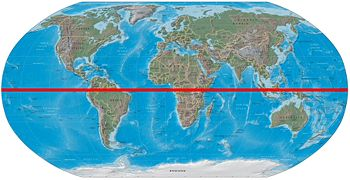# A Line That Divides The Earth Into Two Equal Halves (two Words). Can You Help?

The answer is the Great Circle.  Thanks all for your help everyone

thanked the writer.
The prime meridian divides the earth into equal halves, but that's 13 letters.  Any line of longitude will too, for that matter.  The equator does, but it's only one word.
thanked the writer.
Cool I have the same crossword but I'm in 6th grade mi teacher told mi it was great circle
thanked the writer.

Any line around the earth that divides it into two halves is a GREAT CIRCLE.

thanked the writer.
Is it the Continental Divide?
thanked the writer.
I would say it is the equator
thanked the writer.
I would say prime meridian.
thanked the writer.
It is called the equator
thanked the writer.
Great Circle
thanked the writer.
Southern and Northern Hemispheres
thanked the writer.
The arctic circle
thanked the writer.
Prime meridian
thanked the writer.
The great circle?
thanked the writer.
Equator
thanked the writer.
Prime meridian
thanked the writer.
Equatorial line.
thanked the writer.
That is an imaginary line that divides the northern and southern hemisphere halfway between the poles.

It is called The Equater Line.
thanked the writer.
I think they call it the prime meridian.
thanked the writer.
Hemispheres? Longitude lines? Equator? Prime meridian? Latitude lines?
thanked the writer.
The equator will, or any longitudinal line, including the prime meridian.

thanked the writer.
Hemispheric
thanked the writer.
Prime meridian
thanked the writer.
Prime meridian
thanked the writer.
Prime meridian
thanked the writer.
Hemisphere.
thanked the writer.
The (imaginary) line that divides the earth into two halves is called the equator.
thanked the writer.
Lines of latitude and longitude do not necessarily divide the earth into two equal halves. The correct answer is: The equator

the second word is: The polar axis
thanked the writer.
Its Hemisphere.People also write it as Hemi-sphere.it is the line that divides the earth into two parts.There are also 11 letters in it!(in greek terminology it means "Half of the sphere).So I hope you got the answer~! :)
thanked the writer.
The Prime Meridian divides the Earth from the North to South Poles, The Equator divides the Earth into two hemispheres.
thanked the writer.
It's the equator. It's an imaginary line that divides the earth into two halves. Check out the uploaded image.thanked the writer.